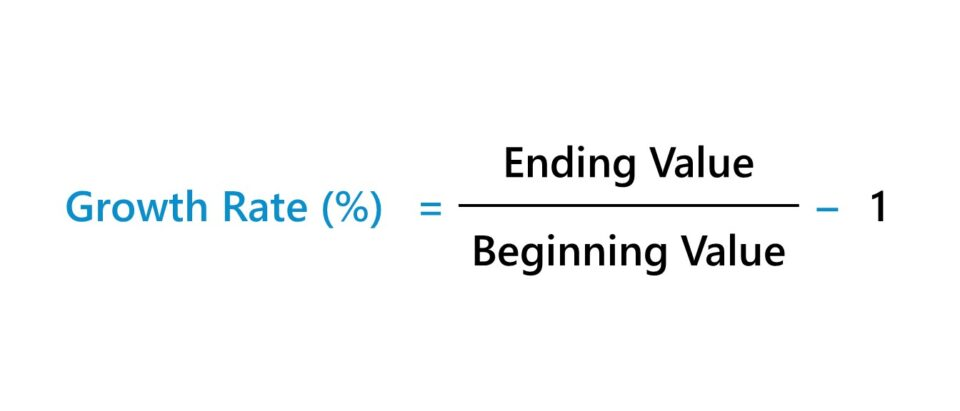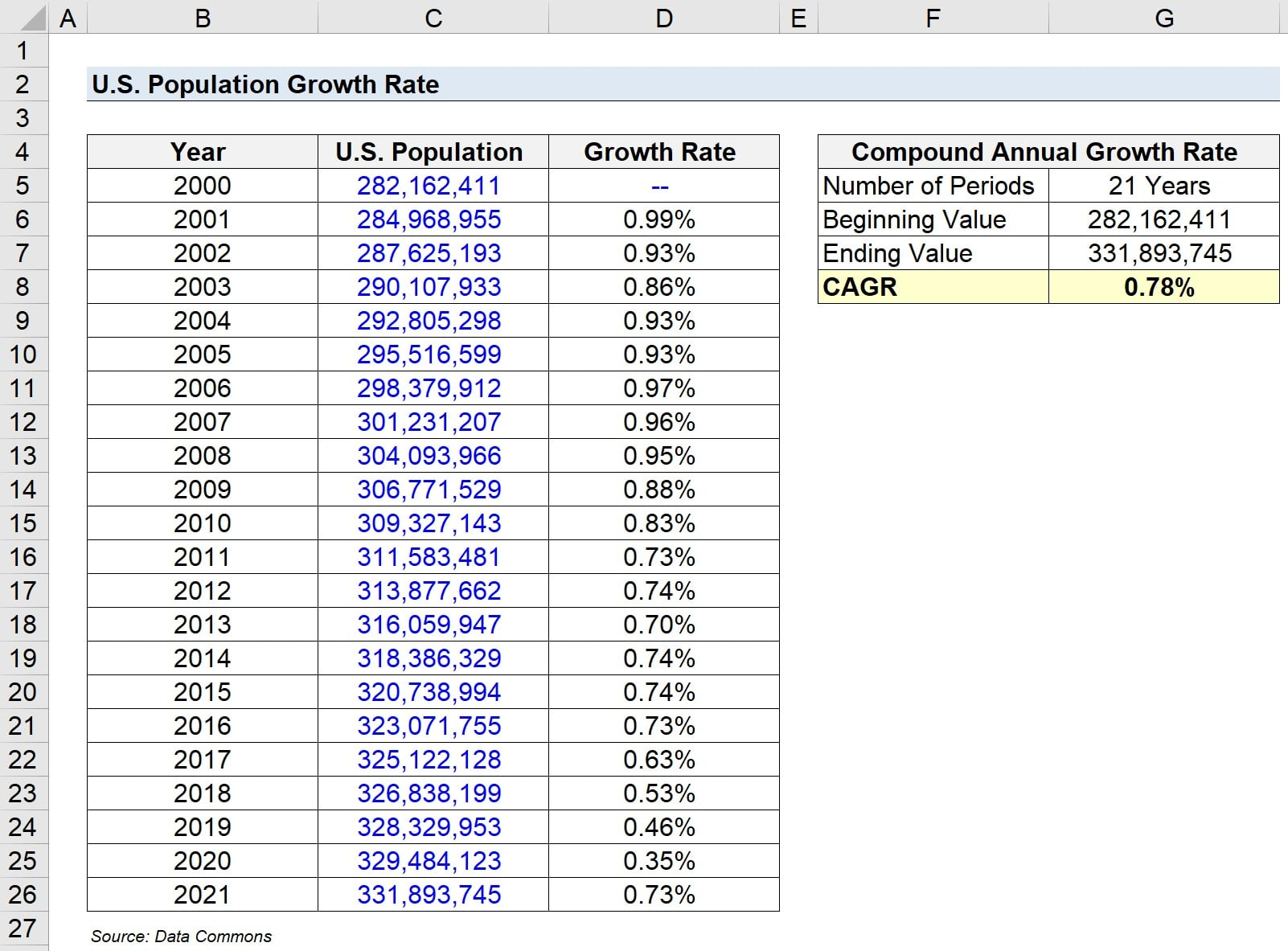Welcome to Wall Street Prep! Use code at checkout for 15% off.# Growth Rate

Guide to Understanding the Growth RateHow to Calculate Growth Rate?

The term “growth rate” describes the rate of change in the value of a specific metric across a given time period, expressed as a percentage.

Common examples of scenarios where the growth rate is often used are the following:

• Company Sales (or Revenue)
• Population Figures
• Gross Domestic Product (GDP)
• Inflation Rate (CPI)

Under the specific context of financial modeling, the growth rate is most frequently on a quarterly or annual basis, i.e. year-over-year (YoY).

More defensible predictions can be made about the future trajectory of a metric in question by determining its historical growth, which can serve as a practical point of reference for forecasting purposes.

However, the metric’s usefulness is still tied to the extent that the underlying drivers are identified and researched in-depth.

By itself, calculating the historical growth is not enough, because what actually caused the past growth and which qualitative factors are likely to determine a metric’s future growth must also be clearly understood.

## Growth Rate Formula

The following formula can be used to calculate the growth rate across two periods.

Growth Rate (%) = (Ending Value ÷ Beginning Value) 1

For example, if a company’s revenue was \$100 million in 2020 and grew to \$120 million in 2021, its year-over-year (YoY) growth rate is 20%.

• Growth Rate = (\$120 million ÷ \$100 million) – 1 = 0.20, or 20%

## How to Annualize the Growth Rate?

Often, we’ll want to annualize the rate of growth (i.e. “smooth out” the rate of growth across several periods), which can be done by calculating the compound annual growth rate (CAGR).

The CAGR is the annualized growth rate over a specified period, i.e. a single growth rate that treats all the past changes as if they occurred evenly across the entire duration of time covered.

CAGR is conceptually the annual rate of return needed for the initial value of a metric to grow from its current value to its ending value between the two specified dates.

The three inputs necessary to compute the CAGR are listed below.

1. Beginning Value
2. Ending Value
3. Number of Periods

Calculating the CAGR involves dividing the ending value by the beginning value, raising the resulting figure to the inverse number of periods (1 ÷ the number of periods), and subtracting one.

CAGR = (Ending Value ÷ Beginning Value) ^ (1 ÷ Number of Periods) 1

Note: The number of periods refers to the number of compounding periods.

## Growth Rate Calculator

We’ll now move to a modeling exercise, which you can access by filling out the form below.#### Get the Excel Template!Submitting...

## 1. U.S. Population Statistics Assumptions

Suppose we’re attempting to evaluate the growth of the U.S. population.

The data set we’re working with – pulled from Data Commons – can be found below.

Year U.S. Population
2000 282,162,411
2001 284,968,955
2002 287,625,193
2003 290,107,933
2004 292,805,298
2005 295,516,599
2006 298,379,912
2007 301,231,207
2008 304,093,966
2009 306,771,529
2010 309,327,143
2011 311,583,481
2012 313,877,662
2013 316,059,947
2014 318,386,329
2015 320,738,994
2016 323,071,755
2017 325,122,128
2018 326,838,199
2019 328,329,953
2020 329,484,123
2021 331,893,745

Source: Data Commons

## 2. Growth Rate Calculation Example

To calculate the year-over-year (YoY) growth rate, we’ll divide each year by the preceding year.

For example, the formula for calculating the YoY growth in 2001 is the current population in 2021 (284,968,955) divided by the population in 2000 (282,162,411), minus one.

• 2001 YoY Growth = (284,968,955 ÷ 282,162,411) – 1 = 0.99%

The growth rates for each year are as follows.

• 2000 → 2001 = 0.99%
• 2001 → 2002 = 0.93%
• 2002 → 2003 = 0.86%
• 2003 → 2004 = 0.93%
• 2004 → 2005 = 0.93%
• 2005 → 2006 = 0.97%
• 2006 → 2007 = 0.96%
• 2007 → 2008 = 0.95%
• 2008 → 2009 = 0.88%
• 2009 → 2010 = 0.83%
• 2010 → 2011 = 0.73%
• 2011 → 2012 = 0.74%
• 2012 → 2013 = 0.70%
• 2013 → 2014 = 0.74%
• 2014 → 2015 = 0.74%
• 2015 → 2016 = 0.73%
• 2016 → 2017 = 0.63%
• 2017 → 2018 = 0.53%
• 2018 → 2019 = 0.46%
• 2019 → 2020 = 0.35%
• 2020 → 2021 = 0.73%

## 3. Compound Annual Growth Rate Calculation Example (CAGR)

Next, the inputs used to calculate the compound annual growth rate (CAGR) are as follows.

• Number of Periods = 21 Years
• Beginning Value = 282,162,411
• Ending Value = 331,893,745

The CAGR of the U.S. population from 2000 to 2021 comes out to 0.78%.

• CAGR = (331,893,745 ÷ 282,162,411) ^ (1 ÷ 21 Years) – 1 = 0.78%Step-by-Step Online Course

### Learn Buy-Side (Hedge Fund) Modeling

Led by a former hedge fund PM (Maverick, Citadel, DE Shaw, Schonfeld), this program begins where financial modeling training ends — with a deep-dive into how buy-side analysts build financial models to make key investment decisions.

Inline Feedbacks